Courses

# NEET Previous Year Questions (2014-20): Chemical Kinetics Notes | EduRev

## NEET : NEET Previous Year Questions (2014-20): Chemical Kinetics Notes | EduRev

The document NEET Previous Year Questions (2014-20): Chemical Kinetics Notes | EduRev is a part of the NEET Course Chemistry Class 12.
All you need of NEET at this link: NEET

Q.1. The rate constant for a first-order reaction is 4.606 × 10-3 s-1. The time required to reduce 2.0 g of the reactant to 0.2 g is:     (2020)
A: 500 s
B: 1000 s
C: 100 s
D: 200 s
Ans:
A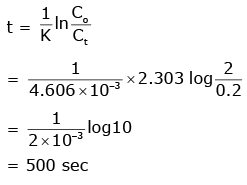Q.2. If the rate constant for a first-order reaction is k, the time (t) required for the completion of 99% of the reaction is given by:    (2019)
A: t = 0.693/k
B: t = 6.909/k
C: t = 4.606/k
D: t = 2.303/k
Ans:
C
The first-order rate constant is given as,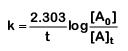99% completed reaction,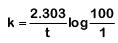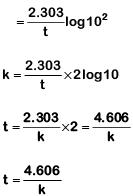Q.3. For the chemical reaction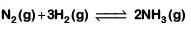The correct option is:    (2019)
A: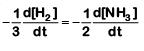B: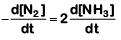C: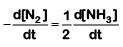D:Ans:
C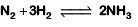The rate of reaction is given as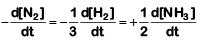Q.4. When the initial concentration of the reactant is doubled, the half-life period of a zero-order reaction    (2018)
A: is halved
B: is doubled
C: is tripled
D: remains unchanged
Ans:
B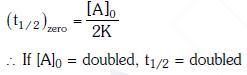Q.5. The correct difference between first- and second-order reaction is that    (2018)
A: the rate of a first-order reaction does not depend on reactant concentration; the rate of a second-order reaction does depend on reactant concentrations.
B: the half-life of a first-order reaction does not depend on [A]0; the half-life of a second-order reaction does depend on [A]0
C: a first-order reaction can be catalyzed; a second-order reaction cannot be catalyzed.
D: the rate of a first-order reaction does depend on reactant concentrations; the rate of a second-order reaction does not depend on reactant concentrations
Ans:
B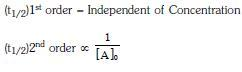Q.6. Mechanism of a hypothetical reaction    (2017)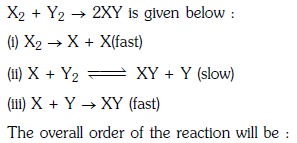A: 2
B: 0
C: 1.5
D: 1
Ans: C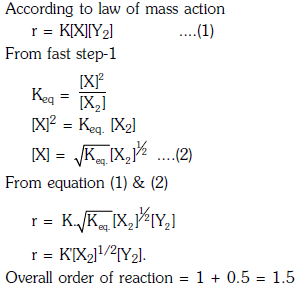Q.7. A first-order reaction has a specific reaction rate of 10–2 sec–1. How much time will it take for 20g of the reactant to reduce to 5 g ?    (2017)
A: 138.6 sec
B: 346.5 sec
C: 693.0 sec

D: 238.6 sec
Ans:
A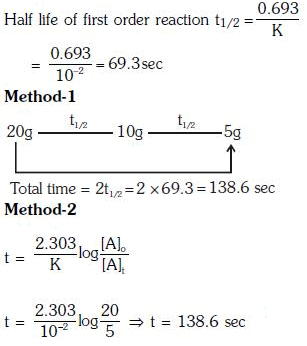Q.8. The rate of a first-order reaction is 0.04 mol ℓ-1 s-1 at 10 seconds and 0.03 mol ℓ-1 s-1 at 20 seconds after initiation of the reaction. The half-life period of the reaction is :    (2016)
A: 54.1 s
B: 24.4 s
C: 34.1 s
D: 44.1 s
Ans:
B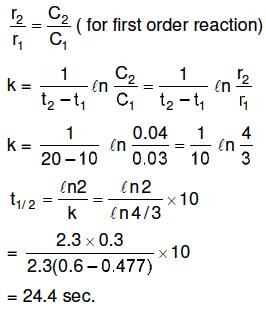Q.9. The activation energy of a reaction can be determined from the slope of which of the following graphs?    (2015)
A: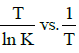B:
ln K vs. T
C: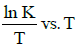D: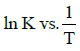Ans:
D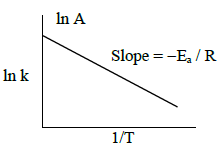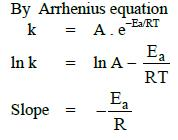Q.10. When the initial concentration of a reactant is doubled in a reaction, its half-life period is not affected. The order of the reaction is :    (2015)
A: More than zero but less than first
B: Zero
C: First
D: Second
Ans:
C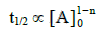For the first-order reaction, the half-life period does not depend upon initial concentration.

Offer running on EduRev: Apply code STAYHOME200 to get INR 200 off on our premium plan EduRev Infinity!

## Chemistry Class 12

106 videos|246 docs|198 tests

,

,

,

,

,

,

,

,

,

,

,

,

,

,

,

,

,

,

,

,

,

;